1. Home >
2. Apps >
3. Groups >

# Solve the series: 13,19=>4 ...

Question asked by pavani abburi in #Coffee Room on Dec 11, 2012pavani abburi · Dec 11, 2012
Rank D2 - MASTER
13,19=>4
.......

71,9=>10

then

128,32=>?

. Posted in: #Coffee RoomKaustubh Katdare · Dec 11, 2012
Rank A1 - PRO
13 + 19 = 32 | 8 x 4 = 32.

71 + 9 = 80 | 8 x 10 = 80

128 + 32 = 160 | 8 x 20 = 160Rising star · Apr 25, 2013
Rank D3 - MASTER
20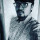Karshil Sheth · Jan 26, 2020
Rank C2 - EXPERT

Add 13+19=32, 71+9=80, 128+32=160. So now if the answers to following question is 4,10 then for the third one with respect with addition it can be 20. [ logical as 4*8=32, 10*8=80, 20*8=160].

So 128,32=>20.

Hope its right?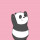Divisha Madupalli · Feb 14, 2020
Rank C1 - EXPERT

20.

13+19=32

71+9=80

128+32=160

all of the sums are multiples of 4 so divide them and see the logic.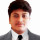Swarup Pan · Feb 17, 2020
Rank C2 - EXPERT

The answer for this question will be 20.

The sum of the first two numbers is a multiple of 8.

By this logic you can easily calculate the answer as 20.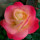supriya VN gowda · Feb 27, 2020
Rank C2 - EXPERT

The answer for these question will be 20.

Add both numbers and check if that number is multiple of 8.

13+19=32.     8*4=32

71+9=80         8*10=80

128+32=160   8*20=160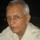Ramani Aswath · Feb 28, 2020
Rank A1 - PRO

There can be other interpretations. The data is too small.

Just to be cantankerous

13,19 => 4    (13,19  so 3+1=4)

71,9=>10      (71,9    so 1+9=10)

128,32=>11    (128,32 so 8+3=11)Venkat Sai Nakkina · Mar 11, 2020
Rank C3 - EXPERT

13+19=32 which is 4*8

71+9=80 which is 10*8

128+32=160 which is 20*8

All are multiples of 8 so the answer is 20

## You must log-in or sign-up to reply to this post.

Click to Log-In or Sign-Up